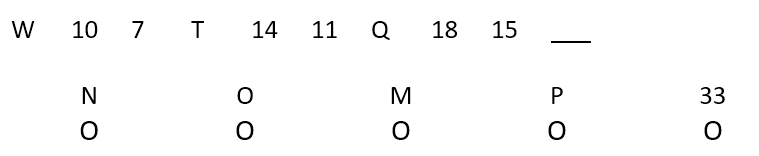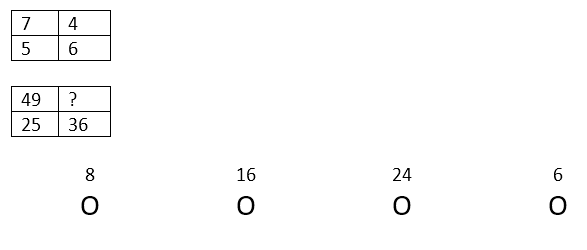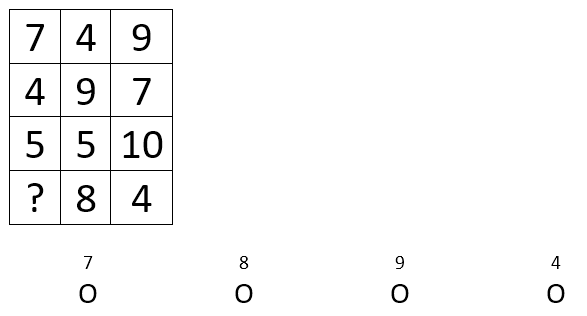Start Now with 100 Free Questions

# OLSAT Quantitative Reasoning

This section of the OLSAT test measures the logical comprehension of the relationship between numbers and numeric groupings, complete number sequences and series as well as the computational abilities in context, to derive at a particular answer. These questions require that students be able to predict outcomes based on their knowledge of mathematics. Quantitative Reasoning consists of number series, numeric inference and number matrix.

Number Series questions assess the ability to understand the pattern in a sequence of numbers or letters and the ability to fill in a missing item in the series. Numeric Inference questions test the ability to understand relationships between numbers and number groupings. Number Matrix questions assess the ability to fill in the missing number in a matrix made up of entirely of numbers. Matrices are 3X3 grids (9 total boxes) which have sequences and patterns that run horizontally and vertically. Each matrix will have a blank square, which will have to be filled in with the correct number to complete the pattern.

## Number Series

In the number series questions, students must examine a sequence of numbers and then determine a pattern that governs those numbers. That pattern is then applied in order to predict what comes next in sequence. Number Series is on levels D-G of the OLSAT, which covers 3rd grade through 12 Grade.

### Sample Question:

Select the number or letter that belongs in the empty space.Answer:  1 – Three letters back each time – Level D

## Numeric Inference

Using computational skills, students need to determine how two or three numbers are related. After they determine this relationship, they then apply this rule to another pair or trio of numbers. Numeric Inference is on levels D-G of the OLSAT, which covers 3rd grade through 12 Grade.

### Sample Question:

The following numbers go together by following the same rule. Determine the rule and find the next number.Answer:  2 – number x itself – Level E

## Number Matrix

Students examine numbers in a matrix and determine what principle or rule links those numbers. Then, this rule applied to figure out what number should be placed in a given blank. Number Matrix is on levels D-G of the OLSAT, which covers 3rd grade through 12 Grade.

### Sample Question:

Find the pattern of the rows in the table and determine the missing number.Answer:  2 – all rows equal 20 – Level E